f
Neutrium

# Fluid-flow

Schmidt Number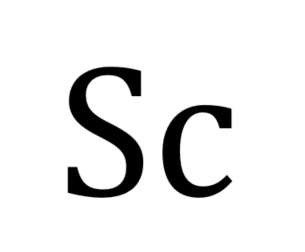The Schmidt number is a dimensionless number that describes the ratio of momentum diffusivity to mass diffusivity that is commonly used in analysis of mass transfer systems. This article describes the Schmidt Number and typical formulations.

Cv versus Orifice SizeThe pressure drop or flow rate through a valve or orifice plate is typically calculated using the a flow coefficient, Cv or orifice diameter. This article demonstrates how to convert between these two parameters when performing functions such as selecting a valve with an equivalent pressure drop to a given orifice plate.

Joule-Thomson Effect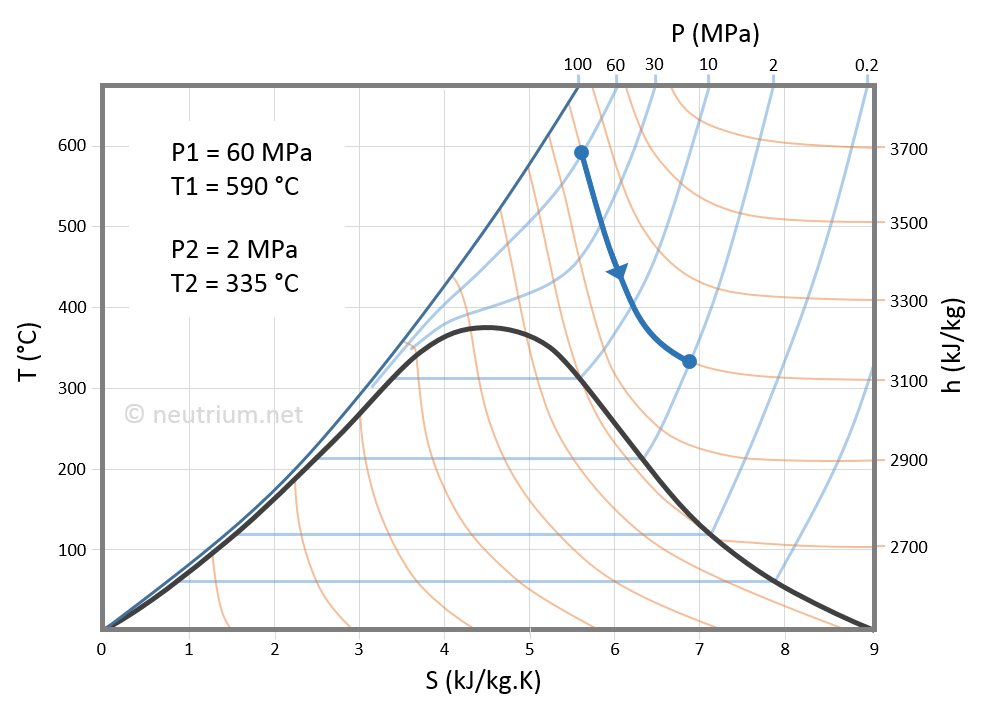The Joule-Thomson Effect describes the change in temperature of a gas as it experiences a rapid change in pressure from passing through a valve, orifice or nozzle. It may represent a safety hazard, or an opportunity depending on the process.

Choked Flow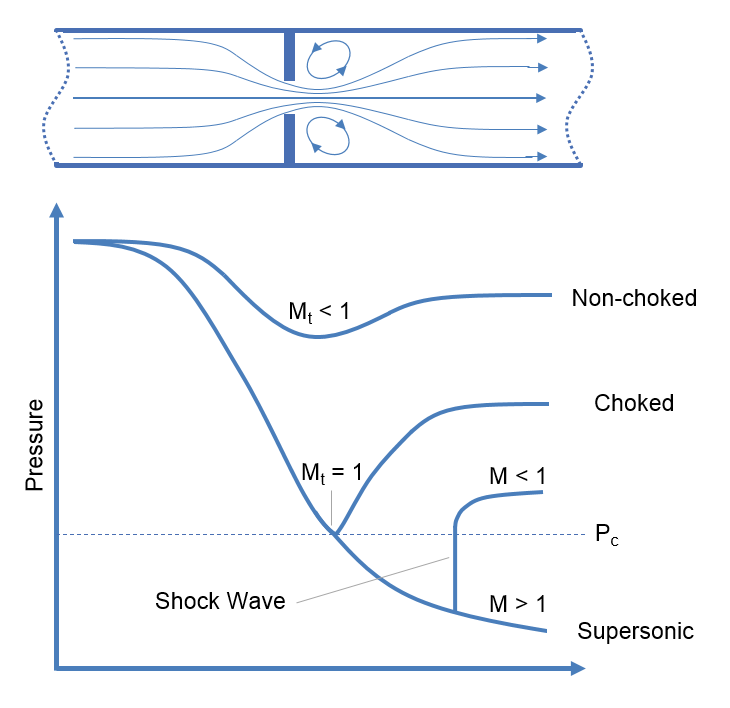Choked flow is a phenomenon that limits the mass flow rate of a compressible fluid flowing through nozzles, orifices and sudden expansions. Generally speaking it is the mass flux after which a further reduction in downstream pressure will not result in an increase in mass flow rate.

Erosion Velocity for Gas-Liquid Multiphase Flow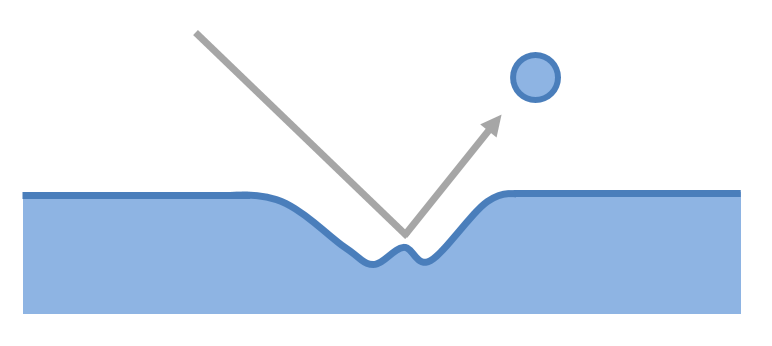The flow of a gas-liquid multiphase system may cause erosion if velocities are high. This article presents an empirical relationship for estimating whether erosion will occur in a system at a certain velocity.

Discharge Coefficient for Nozzles and Orifices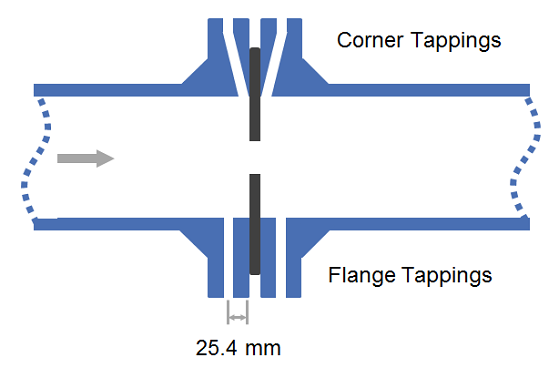The discharge coefficient is a dimensionless number used to characterise the flow and pressure loss behaviour of nozzles and orifices in fluid systems. Orifices and nozzles are typically used to deliberately reduce pressure, restrict flow or to measure flow rate. This article gives typical values of the discharge coefficient for common orifice and nozzle designs.

Calculation of Flow through Nozzles and Orifices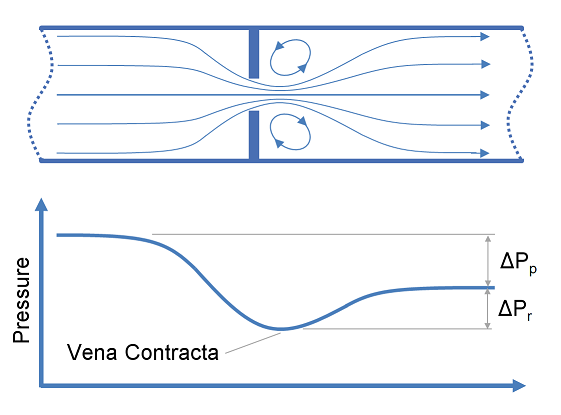This article provides calculation methods for correlating design, flow rate and pressure loss as a fluid passes through a nozzle or orifice. Nozzles and orifices are often used to deliberately reduce pressure, restrict flow or to measure flow rate.

Joukowsky Equation

The Joukowsky equation is a method of determining the surge pressures that will be experienced in a fluid piping system. When a fluid in motion is forced to either stop or change direction suddenly a pressure wave will be generated and propagated through the fluid. This pressure wave is commonly referred to as fluid hammer (also known as water hammer, surge or hydraulic shock) and typically occurs in piping systems when a valve is suddenly closed, isolating the line. The resultant surge pressures are complex to characterise but for simple systems they may be calculated using the Joukowsky equation.

Manning Characteristic RoughnessThe Manning Characteristic Roughness is used to characterise the surfaces over which water can flow in streams, channels, ditches and flumes. This article presents a reference of roughness values for many common materials of construction for channels and natural formations of streams.

Flow in Open Channels and Partially Filled Pipes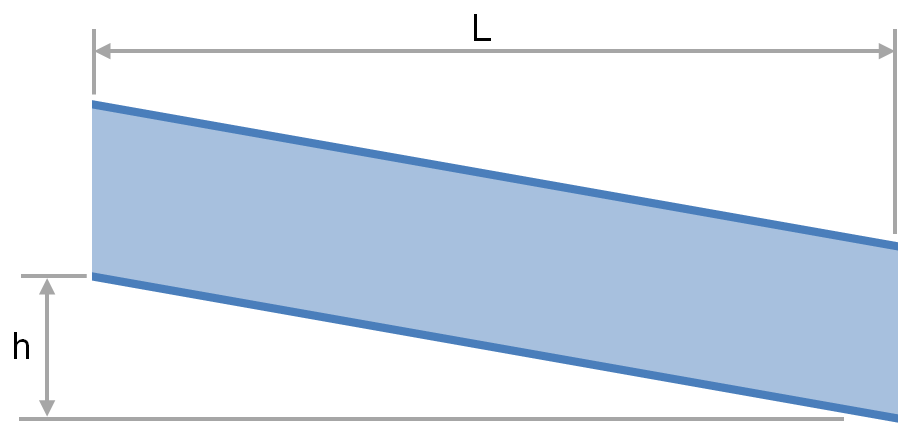The transport of fluid under gravity is often achieved using partially filled pipes, channels, flumes, ditches and streams. To determine the slope and elevation change required or the flow rate that is achievable one must be able to calculate the head loss and friction factor. This article provides relationships for the calculation of head loss and friction factor for fluids flowing via these conduits.